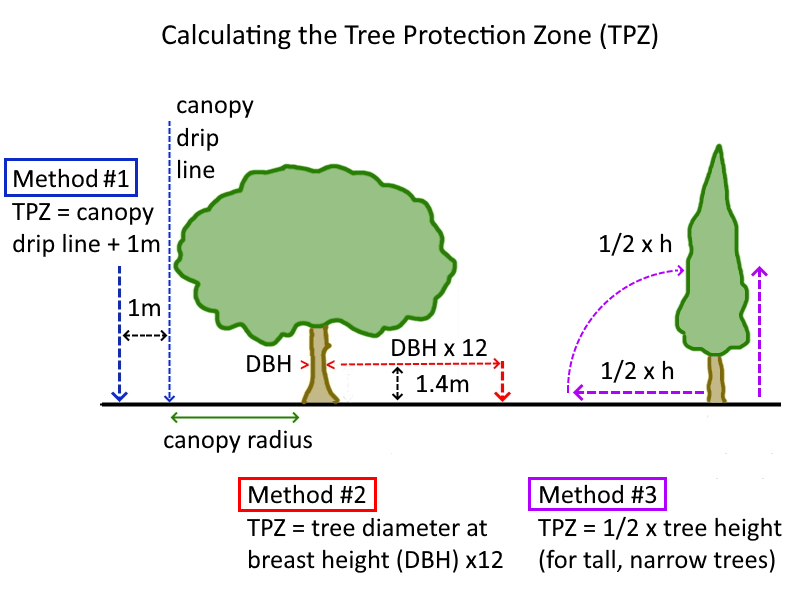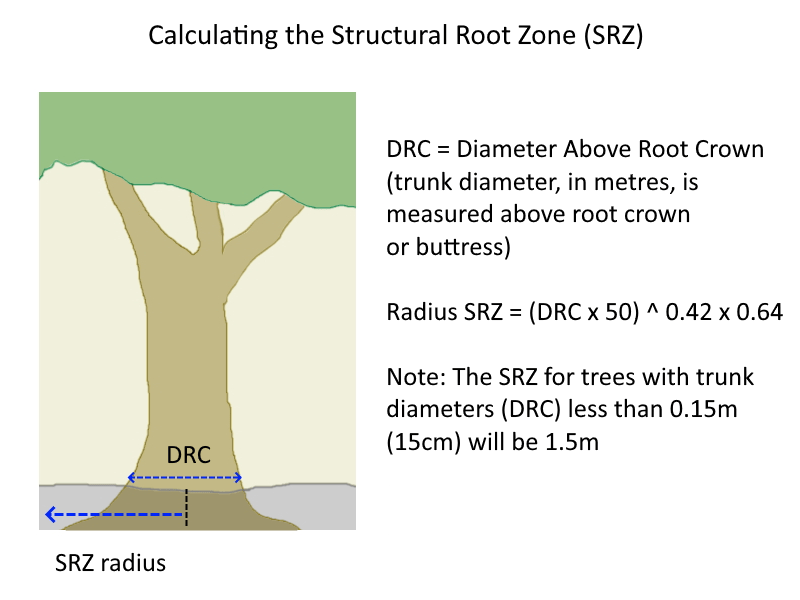# How Far Do Large Tree Roots Extend?

Arboriculture is the cultivation, management, and study of trees and shrubs, and arborists use several formulas for calculating how far tree roots extend in order to protect the health of trees when construction works and soil disturbance occurs nearby.

Knowing how far tree roots extend can be important for locating large shade trees so their roots don’t encroach into nearby garden beds and affect the growth of the plants there by taking up their water and nutrients.

It’s also important to be able to work out where tree roots can be cut into when installing root barriers, without killing the tree or causing it to fall over!

## How to Calculate Tree Root Spread

Calculating how far tree roots reach out is fairly straightforward.

Most tree roots spread 2-3 times the radius of the canopy, and often reach out 5 times the radius of the tree canopy or more in dry conditions.

So, for example, if a tree is 6m wide, the radius of the canopy is 3m.

The root spread = 2 (to 3) x canopy radius = 2 (to 3) x 3m = 6m (to 9m).

Therefore the roots will radiate out from the trunk to a distance of 6m to 9m, and up to 5 x 3m =15m!

So if you’re wondering why your newly planted trees aren’t growing, and the neighbour’s giant trees are within that root range, then there’s the answer. Large tree roots can extend considerable distances, taking up water and nutrients that young trees need to grow. Installing a root barrier along the fence line can give new trees a chance be reducing competition from large trees nearby.

You can use this formula to determine how far any nearby tree roots might reach before digging a hole to plant a new tree or selecting a location for a new garden bed.

## What is the Tree Protection Zone (TPZ)

The Tree Protection Zone (TPZ) is an area defined by arborists as being off limits to any construction because of the location of roots that are critical to tree health.

The TPZ indicates the zone that should be protected from development on all sides if a tree is to be retained, and only on an arborist’s approval can construction encroach on this area, as root damage will lead to drought stress and possibility the death of the tree, and the effects of such damage may take several years to show in the crown of the tree.

## How to Calculate the Tree Protection Zone (TPZ) DistanceThree of the most common methods for calculating the Tree Protection Zone (TPZ) around a tree

There are three conventions for calculating the Tree Protection Zone (TPZ):

1. TPZ = canopy drip line + 1m
This is a less accurate method, where the edge of the tree canopy (known as the canopy drip line) is identified, and extended out one metre further to determine the boundary of the TPZ.
2. TPZ = DBH x 12 , where DBH (Diameter at Breast Height) = trunk diameter measured at 1.4 m above ground
This is a more commonly used method, where the radius of the TPZ is calculated for the tree by multiplying its trunk width (diameter) at breast height, 1.4m above the ground (DBH) and multiplying it by 12.
Note, the radius is measured from the centre of the stem at ground level.
3. TPZ = 1/2 x Height
This method is only used for tall, narrow trees, the TPZ is defined as extending out to half the tree height.

Note: Section 3.2 of the Australian Standard AS4970–2009 (Protection of Trees on Development Sites) states that the TPZ of palms and other monocots, cycads and tree ferns (pachycauls) should not be less than1 m outside the crown projection (canopy drip line).

## What is the Structural Root Zone (SRZ)

The Structural Root Zone (SRZ) is an area around the tree trunk which is essential for tree stability. Damage to the roots in this area will most likely cause the tree to become unstable in the ground, which means the tree can possibly fall over.

The TPZ, which is essential to tree health, is a larger area than the SRZ, which is important for tree stability.

If construction or digging will intrude significantly into the Tree Protection Zone (TPZ), the Structural Root Zone (SRZ) is calculated to ensure that the works will not cause the tree to become unstable and fall over.

## How to Calculate the Structural Root Zone (SRZ) DistanceThe Structural Root Zone (SRZ) area is critical to tree stability, it prevents the tree from falling over!

Calculating the Structural Root Zone (SRZ) is a bit more complicated.

The DRC (Diameter Above Root Crown) is the width (diameter) of the tree trunk measured immediately above the root crown or root buttress.

The formula for the Structural Root Zone (SRZ) radius is as follows:

SRZ radius = (DRC x 50)^0.42 x 0.64

Where

• DRC (Diameter Above Root Crown) = trunk diameter, in metres, measured above root crown
• Radius is measured from the centre of the stem at ground level.

To calculate, multiply the DRC value by 50, then multiply the result by the power of 0.42 (which is not the same as simply multiplying it by 0.42), and then multiply the result by 0.64.

If using Microsoft Excel to calculate the formula, type it in as =(DRC*50)^0.42*0.64
(where DRC is your measurement of the width of the tree above the crown)

For example, if the DRC is measures to be 0.6m, the formula in Microsoft Excel should be types as follows:

=(0.6*50)^0.42*0.64

This example will return a value of 2.67m if typed correctly.

Note: The SRZ for trees with trunk diameters (DRC) less than 0.15 m (15cm) will be 1.5 m.

The SRZ formula is not used for palms, other monocots, cycads & tree ferns. Also, pachycauls – trees with thick, fat stems/trunks with few branches, such as Baobabs and Brachychitons technically do not conform to the definition of trees according to arborists, and therefore do not technically have a SRZ, though in the real world are trees and do have structural roots!

## How Far from the Tree Should Tree Root Barriers be Installed?

Tree root barriers should be installed outside of the SRZ to maintain tree stability.

A simple formula for estimating the minimum distance that tree root barriers can be placed is as follows:

Minimum distance to root barrier = 3.5 x DBH

Where

• Minimum distance is measured from the centre of the stem to the root barrier
• DBH (Diameter at Breast Height) = trunk diameter measured at 1.4 m above ground when mature.

Note: The minimum distance should be 1.5m if the calculated figure is less than this.

1.martin@muchmoremulch.blog says:

A very insightful and informative article, thank you Angelo. What I’m interested in is the required depth of the root barrier? E.g. Can roots grow down and below and up again on the other side of a root barrier? How deep should you dig in a root barrier to be effective? (I guess it will depend on the type of tree and the shape and depth its roots – so if want to, lets talk about that big water sucking Eucalyptus…)

1.Angelo (admin) says:

Root barriers are usually 600mm deep as most tree roots would be stopped at this depth. Some really invasive trees have roots that run deeper, which is why 900mm high root barrier is also available.Many trees have root systems comprised mainly of fine surface roots, and even a 300mm deep barrier will halp a little, though roots will eventually grow under.

1.martin@muchmoremulch.blog says:

Thanks Angelo – much appreciated 🙂

2.Alena Klime�ov� says:

Hello, thank you for the article. Can you please tell me what is the source of the SRZ calculation. It will help me with my doctoral studies. Thanks a lot.

1.Angelo (admin) says:

The source of the SRZ calculation formula source is the Australian Standard related to arboriculture – Protection of Trees on Development Sites (AS 4970-2009)

3.RMK says:

Hi Many thanks for your publication
We have an Adasonia digitata (baobad tree) and we requiere relocate it.

Its DRC is 0.4 m, an by your publication i did calculate the SRZ radius on 2 m.

I’m worry about the size of the root ball i should keep during the relocation. Tree height is about 4m. The DBH is 0.14. Canopy radius is about 1.8m.

How i can estimates an apptopiated root ball size?

1.Angelo (admin) says:

Adansonia digitata, the African baobab tree produces an extensive lateral root system until 50 m from the trunk. The roots tips are often in the form of tubers. But the main roots of old trees are relatively shallow and rarely extend beyond 2 m depth. Therefore they are very sensitive to strong winds and can be uprooted by storm. (From https://www.sciencedirect.com/science/article/pii/S222116911530174X)

The baobab tree has such a unique root system, most of the roots spread sideways, but for a young tree, using a 2m radius for the SRZ probably sounds about right, this will cover a 4m wide circle of roots, which hopefully encompasses a lot of the lateral root spread. It might be worth doing some exploratory digging to see if there are any tubers at the end of the root tips, and if there are, how far they are from the tree, as you would want to keep these intact when transplanting.

Please let us know how the transplant goes, how far the roots go, and if the transplant succeeded, this is valuable knowledge as they are now becoming endangered species! Thanks